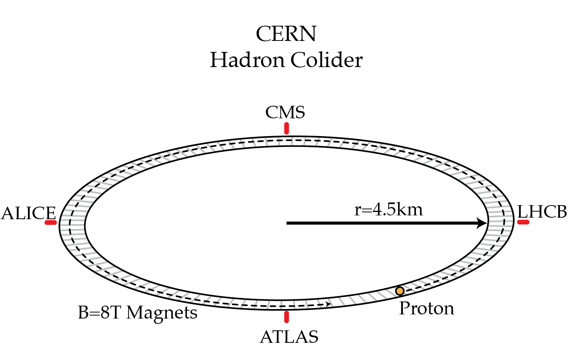# Zipping round and round

The Large Hadron Collider at CERN has a radius of $R=4.5~km$ and uses $B=8~T$ magnets to bend the particles around the accelerator. Estimate the energy of protons going around the accelerator in J?The energy of a relativistic particle is calculated by $E=\sqrt{p^2c^2+m^2c^4}$, with $p$ is the particle's momentum, $m$ is the particle's mass and $c$ is the speed of light.

You may want to check this Minute Physics clip for a little background on relativistic momentum.

The proton's speed is extremely close to the speed of light.

Note: we're actually going to come out a touch high for the energy per proton, but it's still a pretty good estimate considering how simply we've modeled the LHC.

Details and assumptions

• The proton charge is $-e=|e|=1.6 \times 10^{-19}~C$ and the speed of light is $c=3 \times 10^{8} ~m/s$. The proton's mass is not necessary in this problem.
×